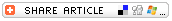#Explain Rounding and EstimatingExplain Rounding and Estimating Whole Number
Rounding is a way of simplifying numbers to make them easier to understand or work with. Rounding can be used when an exact number isn't needed, and an approximate answer will do. For example, at the minute this is being written, exactly 299,588,632 people live in the United States (See the U.S. Census Bureau Population Clock.), but that number changes every day. And it's not likely you'll need to know exactly how many people live in the United States at any given moment. Instead, you could simplify that number by "rounding" it to 300 million. How does that work? Follow the steps below to learn how to round a whole number.

• Find the "round off" digit. The round off digit is the place value of the number you're rounding to. For example, if you want to round a number to the nearest ten, the round off digit is the number in the tens' place. If you want to round a number to the nearest hundred, the round off digit is the number in the hundreds' place.
• Look at the number to the right of the round off digit. If that number is less than 5, don't change the round off digit. If that number is 5 or more, add one to the round off digit.
• Change all the digits to the right of the round off digit to zeros.

Rounding and Estimating Interactive Activities

• Rounding Numbers
• Estimation: Hundreds
• Estimation Contraption
• Rounding Off
• Rounding Flashcards